• 初始化列表
千次阅读
2021-05-07 21:42:36

初始化列表就是一种新型简单的有参构造函数，比以前的构造函数少写一点代码

代码一：

class Person {
public:

传统方式初始化
//Person(int a, int b, int c) {
//	m_A = a;
//	m_B = b;
//	m_C = c;
//}
//初始化列表方式初始化
Person() :m_A(10), m_B(20), m_C(30) {}
void PrintPerson() {
cout << "mA:" << m_A << endl;
cout << "mB:" << m_B << endl;
cout << "mC:" << m_C << endl;
}
private:
int m_A;
int m_B;
int m_C;
};
int main() {
Person p;
p.PrintPerson();
system("pause");
return 0;
}

代码二：

class Person {
public:
传统方式初始化
//Person(int a, int b, int c) {
//	m_A = a;
//	m_B = b;
//	m_C = c;
//}
//初始化列表方式初始化
Person(int a, int b, int c) :m_A(a), m_B(b), m_C(c) {}
void PrintPerson() {
cout << "mA:" << m_A << endl;
cout << "mB:" << m_B << endl;
cout << "mC:" << m_C << endl;
}
private:
int m_A;
int m_B;
int m_C;
};

int main() {
Person p(1, 2, 3);
p.PrintPerson();
system("pause");
return 0;
}

更多相关内容
• 使用初始化列表完成这步操作在性能上有益处。什么好处呢？摆道理显得不够彻底。看书不如做实验。让我们结合执行过程来查看。考虑如下示例代码： 　Derive 类构造函数两个 Base 类型的参数，分别赋给该类两个 ...
• C++ Primer中在讲构造函数初始化列表的时候有这么一段话：无论是在构造函数初始化列表中初始化成员，还是在构造函数体中对它们赋值，最终结果是相同的。不同之处在于，使用构造函数初始化列表的版本初始化数据成员，...
• 今天小编就为大家分享一篇关于C++使用初始化列表的方式来初始化字段的方法，小编觉得内容挺不错的，现在分享给大家，具有很好的参考价值，需要的朋友一起跟随小编来看看吧
• 下面小编就为大家带来一篇关于C++类的成员初始化列表的相关问题。小编觉得挺
• 构造函数初始化列表以一个冒号开始，接着是以逗号分隔的数据成员列表，每个数据成员后面跟一个放在括号中的初始化式。例如： class CExample { public: int a; float b; //构造函数初始化列表 CExample(): a(0...
• 何谓初始化列表与其他函数不同，构造函数除了有名字，参数列表和函数体之外，还可以有初始化列表初始化列表以冒号开头，后跟一系列以逗号分隔的初始化字段。在C++中，struct和class的唯一区别是默认的克访问性不同...
• 构造函数的初始化列表仅仅指定用于初始化成员的值，并不指定这些初始化执行的次序。成员初始化的次序就是定义成员的次序，第一个成员首先被初始化，然后是第二个，依次类推
• 1.只能在构造函数初始化列表初始化的成员变量的类型?  a.const成员变量  b.引用类型的成员变量  c.static不能在初始化列表中进行初始化  d.类成员变量中有自定义类型的变量最好在初始化列表中进行初始化 2.初始...
• 对于类成员是const修饰，或是引用类型的情况，是不允许赋值操作的，(显然嘛，const就是防止被错误赋值的，引用类型必须定义赋值在一起)，因此只能用初始化列表对齐进行初始化。成员类型是没有默认构造函数的类。若...
• C++ 初始化列表 何谓初始化列表 与其他函数不同，构造函数除了有名字，参数列表和函数体之外，还可以有初始化列表初始化列表以冒号开头，后跟一系列以逗号分隔的初始化字段。在C++中，struct和class的唯一区别是...
• 在C++11中，结构体或类的数据成员在申明时可以直接赋予一个默认值，初始化的方式有两种，一是使用等号“=”，二是使用大括号列表初始化的方式。注意，使用参考如下代码： class C { private: int a=7; //C++11 ...
• ## 【C++】-- 初始化列表

千次阅读 多人点赞 2022-04-19 22:36:18
初始化列表：以一个冒号开始，接着是一个以逗号分隔的数据成员列表，每个"成员变量"后面跟一个放在括 号中的初始值或表达式。

目录

一、用初始化列表初始化对象

1.初始化列表用法

2.初始化列表特性

二、explicit关键字

1.内置类型的隐式转换

2.如何避免单参构造函数初始化发生隐式类型转换创建一个类对象时，编译器通过调用构造函数，给类对象中各个成员变量赋初值：

class Date
{
public:
//构造函数
Date(int year = 2022, int month = 4, int day = 19)
{
_year = year;
_month = month;
_day = day;
}

private:
int _year;
int _month;
int _day;
};


但上述赋初值不能称作类对象成员的初始化，因为构造函数体内可以多次赋值：

class Date
{
public:
//构造函数
Date(int year = 2022, int month = 4, int day = 19)
{
_year = year;
_month = month;
_day = day;
_year = 2023;//构造函数体内允许对成员变量进行多次赋值
}

private:
int _year;
int _month;
int _day;
};


而初始化列表能只能初始化一次。

# 一、用初始化列表初始化对象

## 1.初始化列表用法

初始化列表：以一个冒号开始，接着是一个以逗号分隔的数据成员列表，每个"成员变量"后面跟一个放在括 号中的初始值或表达式。

class Date
{
public:
//构造函数
Date(int year = 2022, int month = 4, int day = 19)
:_year(year) //初始化列表初始化
,_month(month)
,_day(day)
{}

private:
int _year;
int _month;
int _day;
};


## 2.初始化列表特性

（1）初始化列表能只能初始化一次，多次初始化会报错：

class Date
{
public:
//构造函数
Date(int year = 2022, int month = 4, int day = 19)
:_year(year)
,_month(month)
,_day(day)
,_month(month) //初始化列表多次初始化
{}

private:
int _year;
int _month;
int _day;
};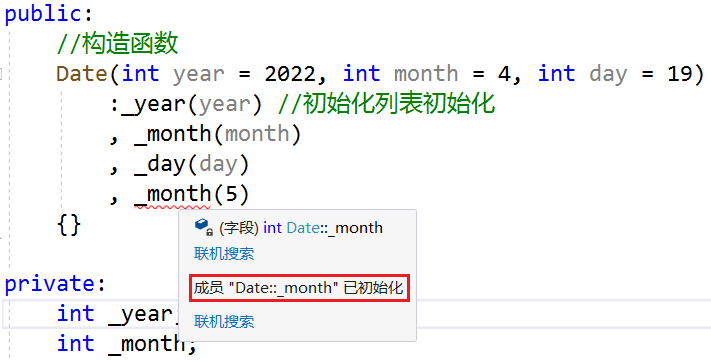编译器也允许构造函数赋初值和初始化列表初始化混用：

class Date
{
public:
//构造函数
Date(int year = 2022, int month = 4, int day = 19)
:_year(year)  //两者混用
,_month(month)
{
_day = day;
}

private:
int _year;
int _month;
int _day;
};


混用时初始化列表初始化和构造函数赋初值不冲突：

class Date
{
public:
//构造函数
Date(int year = 2022, int month = 4, int day = 19)
: _year(year) //两者不冲突
, _month(month)
{
_day = day;
_year = 2023;
}

private:
int _year;
int _month;
int _day;
};


但混用时初始化列表初始化还是要遵循只能初始化一次成员变量的原则：

class Date
{
public:
//构造函数
Date(int year = 2022, int month = 4, int day = 19)
: _year(year) //初始化列表初始化
, _month(month)
, _year(2023)  //_year在初始化列表里被初始化了两次，不允许
{
_day = day;
}

private:
int _year;
int _month;
int _day;
};


（2）const成员变量、引用成员变量、没有默认构造函数的自定义类型成员只能在初始化列表初始化。

①const成员变量必须在定义的时候初始化

class Date
{
public:
//构造函数
Date(int year = 2022, int month = 4, int day = 19)
: _year(year)
, _month(month)
, _n(2) //const成员变量必须使用初始化列表进行初始化
{
_day = day;
//_n = 2; //const成员变量不能在函数体内初始化
}

private:
int _year;
int _month;
int _day;

const int _n = 1;
};

②引用成员变量必须在定义的时候初始化

class Date
{
public:
//构造函数
Date(int year = 2022, int month = 4, int day = 19)
: _year(year)
, _month(month)
,_ref(year)//引用成员变量要在初始化列表初始化
{
_day = day;
//_ref = year; //引用成员变量不能在函数体内初始化
}

private:
int _year;
int _month;
int _day;

int& _ref;
};


③没有默认构造函数的自定义类型成员变量

#include <iostream>
using namespace std;

class A
{
public:

//默认构造函数是不用传参就可以调用的构造函数，有3种：
//1.无参默认构造函数
//2.带参全缺省的默认构造函数
//3.我们不写，编译器自动生成的默认构造函数

A(int x)//不属于以上任何一种，所以A类的对象没有默认构造函数
{
cout << "A(int x)" << endl;
_x = x;
}

private:
int _x;
};

class Date
{
public:
//构造函数
Date(int year = 2022, int month = 4, int day = 19)
: _year(year)
, _month(month)
, _a(20)//没有默认构造函数的自定义类型成员变量必须在初始化列表进行初始化
{
_day = day;
}

private:
int _year;
int _month;
int _day;

A _a;
};


const成员变量、引用成员变量、没有默认构造函数的自定义类型成员变量必须在初始化列表内初始化的原因：

①初始化列表是对象的成员变量定义的地方。

②对象的内置类型成员变量在初始化列表定义时没有要求必须初始化，因此既可以在初始化列表进行初始化，也可以在构造函数体内初始化。

③而const成员变量、引用成员变量、没有默认构造函数的自定义类型成员变量不能先定义再初始化，它们在初始化列表内定义，并且必须在定义时就初始化，因此必须在初始化列表内初始化。

（3） 尽量使用初始化列表初始化，因为不管是否使用初始化列表，虽然对于内置类型没有差别，但是对于自定义类型成员变量，一定会先使用初始化列表初始化。

为什么会先使用初始化列表初始化？

如下，Date类没有默认构造函数，因为26行的构造函数不属于默认构造函数中的任意一种，在对Date类的对象d进行初始化时，会调用Date类的默认构造函数，所以对象d的day实参12和hour实参12都没有被传进去，_t作为Date类的自定义类型成员变量会调用Time类的默认构造函数，_hour默认传参为0，因此打印_hour的值也为0，d的参数没有传成功：

#include<iostream>
using namespace std;

class Date; // 前置声明
class Time
{
friend class Date; // 声明日期类为时间类的友元类，则在日期类中就直接访问Time类中的私有成员变量
public:
Time(int hour = 0)
: _hour(hour)
{
cout << _hour << endl;
}

private:
int _hour;
};

class Date
{
public:
Date(int day, int hour)
{}

private:
int _day;
Time _t;
};

int main()
{
Date d(12, 12);
return 0;
}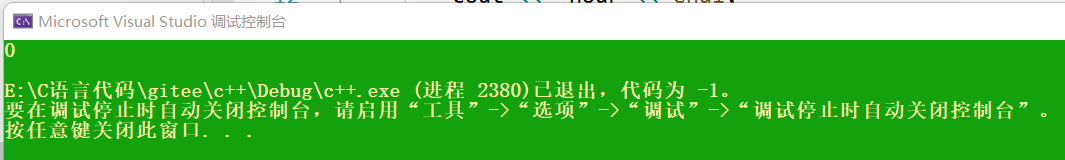假如Date类的构造函数不使用初始化列表进行初始化，使用函数体内初始化时，要把Date类的构造函数的形参hour的值给d，那么就必须构造一个Time类对象t，对该对象传参传hour，再使用赋值运算符重载函数将对象t拷贝给_t：

#include<iostream>
using namespace std;

class Date; // 前置声明
class Time
{
friend class Date; // 声明日期类为时间类的友元类，则在日期类中就直接访问Time类中的私有成员变量
public:
Time(int hour = 0)
: _hour(hour)
{
cout << _hour << endl;
}

private:
int _hour;
};

class Date
{
public:
//自定义类型，不使用初始化列表，就需要使用构造函数 + operator=
Date(int day, int hour)
{
//函数体内初始化
Time t(hour);//调用Time类的构造函数
_t = t;

_day = day;
}

private:
int _day;
Time _t;
};

int main()
{
Date d(12, 12);
cout << 4 << endl;
return 0;
}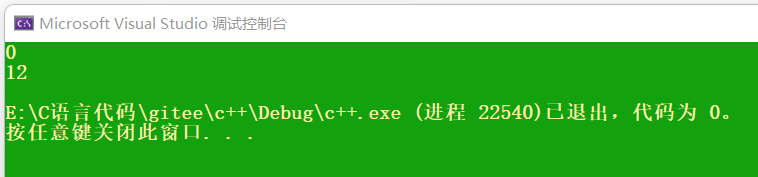这还不如直接使用使用初始化列表初始化呢，还不需要赋值运算符重载函数：

class Date
{
public:
//自定义类型，使用初始化列表，只需要构造函数
Date(int day, int hour)
:_t(hour)
{
_day = day;
}

private:
int _day;
Time _t;
};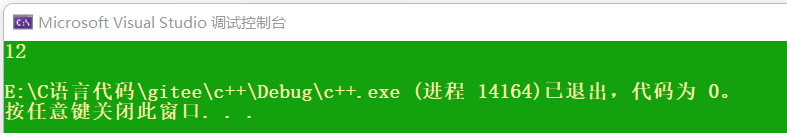因此，建议尽量直接使用初始化列表进行初始化。

（4）成员变量初始化的顺序就是成员变量在类中的声明次序，与初始化列表中的先后次序无关。

如下代码，类成员变量中先声明了_a2，再声明了_a1，因此初始化的顺序是先初始化_a2，再初始化_a1：

#include <iostream>
using namespace std;
class A
{
public:
A(int a)
: _a1(a)
, _a2(_a1)
{}

void Print()
{
cout << _a1 << " " << _a2 << endl;
}
private:
int _a2;//先声明_a2
int _a1;//后声明_a1
};

int main() {
A aa(1);
aa.Print();
}

先声明_a2就会先初始化_a2，用_a1初始化_a2，由于此时_a1还是随机值，因此_a2的值也是随机值，_a1使用a的值1进行初始化，因此，_a1的值为1：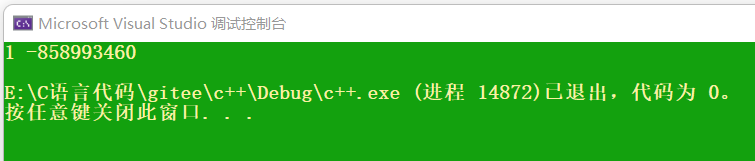所以，建议类中的成员变量声明的顺序和初始化列表中初始化的顺序一致。

# 二、explicit关键字

## 1.内置类型的隐式转换

int i = 0;
double d = i;//隐式类型转换

根据监视可以看出：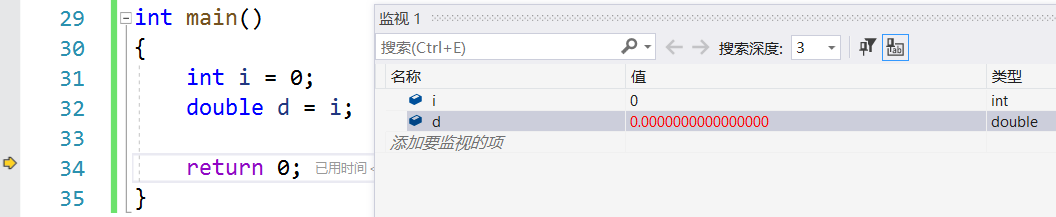double d = i;并不是将i直接赋值给d，而是用i创建一个临时变量，再把临时变量的值给d，那么d改变的是临时变量的值，而不是i的值，因为程序执行完毕后，i的值并未发生改变。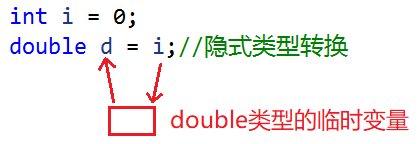如果d作为引用，那么必须加上const关键字进行修饰，因为d不是i的引用，是临时变量的引用，而临时变量具有常性，不允许引用权限放大。

int i = 0;
const double& d = i;//d引用了临时变量，临时变量具有常性，所以d也必须具有常性

## 2.如何避免单参构造函数初始化发生隐式类型转换

正常的类对象初始化如下面的aa1，也可以使用拷贝构造初始化，如aa2。由于c++支持隐式类型转换，因此也支持单参数构造函数初始化，如aa3：

#include<iostream>
using namespace std;

class A
{
public :
A(int a)
:_a(a)
{}

private:
int _a;
};

int main()
{
A aa1(1);//构造aa1对象
A aa2(aa1);//拷贝构造，程序没写拷贝构造，编译器会自动生成拷贝构造函数，对内置类型完成浅拷贝

A aa3 = 3;//单参数的构造函数，会发生隐式类型转换

return 0;
}

那么

A aa3 = 3;

是如何支持类型转换的呢？

对于自定义类型A，aa3是A类型，3是整形。编译器会先拿A构造一个临时对象temp，3作为参数传给这个临时对象temp，再拿aa3(temp)去拷贝构造，发生隐式类型转换，即先构造，再拷贝构造：

//A aa3 = 3;
A temp(3);   //先构造
A aa3(temp); //再拷贝构造

不过现在的编译器已经优化过了，会直接调用构造函数A aa(3)。

如果不想让单参数的构造函数发生隐式类型转换，可以使用explicit关键字修饰构造函数，表明该构造函数是显式的，而不是隐式的，就会避免发生不期望的类型转换，使用场景如下：

#include<iostream>
using namespace std;

class A
{
public:
A(int a)
:_a(a)
{}

private:
int _a;
};

int main()
{
A aa1(1);//构造aa1对象
A aa2(aa1);//拷贝构造，程序没写拷贝构造，编译器会自动生成拷贝构造函数，对内置类型完成浅拷贝

A aa3 = 'x';//先拿A构造一个临时对象temp，字符x作为参数传给这个临时对象temp，会发生隐式类型转换，再拿aa3(temp)去拷贝构造

return 0;
}

aa3作为A类的对象，构造时传参应该传int型，但却传了char型，由于发生隐式类型转换，因此编译也没毛病，但是它传参就是不伦不类。这时候可以给A的构造函数加上explicit声明不让该单参构造函数发生隐式类型转换，编译就会报错：

class A
{
public:
explicit A(int a)
:_a(a)
{}

private:
int _a;
};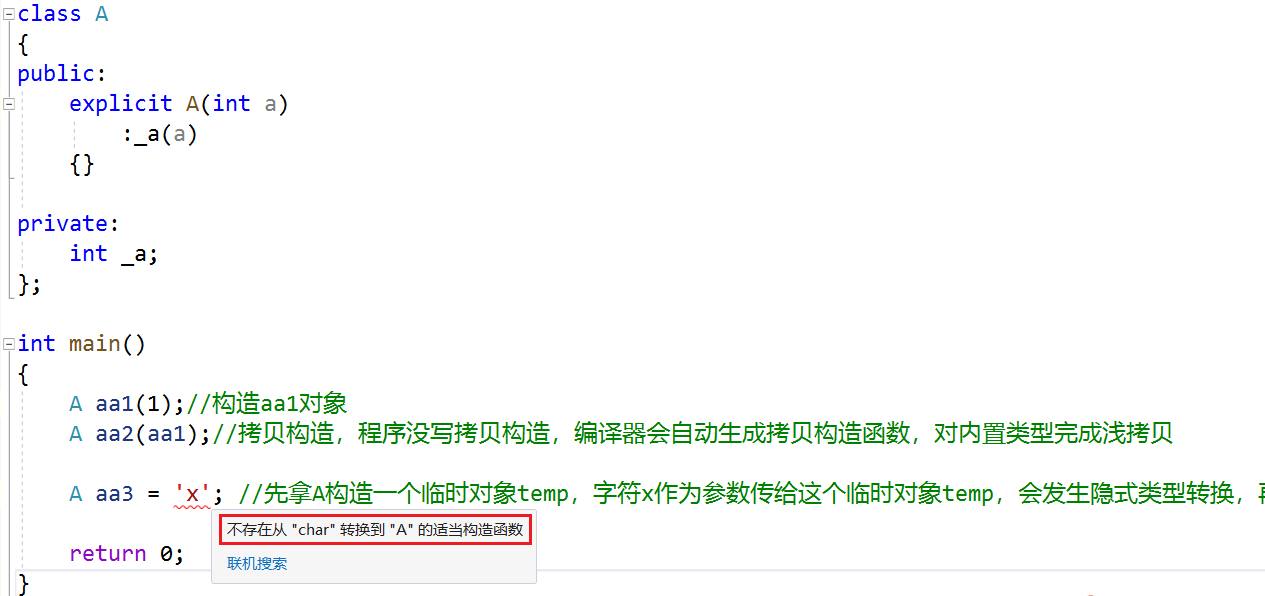这时候只能乖乖给aa3传int型参数了。

# 三、匿名对象

## 1.匿名对象定义

没有名字的对象叫做匿名对象，A(3)跟aa1和aa2相比少了个对象名，没有名字，aa1和aa2的生命周期在main函数内，A(3)的生命周期只在当前行：

#include<iostream>
using namespace std;

class A
{
public:
explicit A(int a)
:_a(a)
{
cout << "A(int a):"<< a << endl;
}

A(const A& aa)
{
cout << "A(const A&)" << endl;
}

~A()
{
cout << "~A()" << endl;
}

private:
int _a;
};

int main()
{
A aa1(1);//生命周期在main函数内
A aa2(aa1);//生命周期在main函数内

A(3);//构造匿名对象，生命周期只在这一行

return 0;
}

F10调试：当执行完A(3)还没执行return 0时，aa1和aa2的生命周期还没有结束，不会调用析构函数，此时打印的析构函数只能是匿名对象A(3)的析构函数：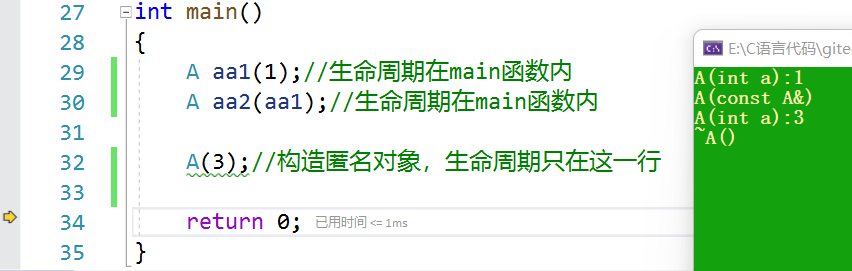所以A(3)这一行执行完就调析构函数了。

## 2.匿名对象应用场景

假设有一个函数f，且A类的构造函数全缺省：

#include<iostream>
using namespace std;

class A
{
public:
A(int a = 0)//构造函数全缺省
:_a(a)
{
cout << "A(int a):"<< a << endl;
}

A(const A& aa)
{
cout << "A(const A&)" << endl;
}

~A()
{
cout << "~A()" << endl;
}

void f()//f函数
{
cout << "f()" << endl;
}

private:
int _a;
};

int main()
{
A aa1(1);//生命周期在main函数内
A aa2(aa1);//生命周期在main函数内

A(3);//构造匿名对象，生命周期只在这一行

return 0;
}

调用f()函数时，需要定义一个A类对象，才能调用A类函数f，这就需要写两行：

int main()
{
A aa1(1);//生命周期在main函数内
A aa2(aa1);//生命周期在main函数内

A(3);//构造匿名对象，生命周期只在这一行

A aa4;//需要定义一个A类对象，才能调用f
aa4.f();

return 0;
}

对象aa4 在main函数结束后才会销毁。如果定义对象只是为了调用函数，那么可以考虑直接定义一个匿名对象：

int main()
{
A aa1(1);//生命周期在main函数内
A aa2(aa1);//生命周期在main函数内

A(3);//构造匿名对象，生命周期只在这一行

A aa4;//需要定义一个A类对象，才能调用f
aa4.f();

A().f();//定义匿名对象来调用函数f()

return 0;
}

这个匿名对象就是为了调用函数f，这个匿名对象后边也没人用它，在当前行调用完f()函数就销毁了。

展开全文开发语言
• 通常layui前端页面完全按照layui官网的...但当你用这个的时候，你会发现列表初始化回显checkbox不生效.这个时候你就怀疑是不是样式有没有加载全或者什么的.其实回到我们刚刚的那个再初始化的方法，这个初始化方法是要在
• ## c++中的初始化列表

千次阅读 2022-04-13 21:52:28
3.最好使用初始化列表进行初始化，因为对于自定义类型，我们还是需要使用初始化列表进行初始化的 4. 成员变量在类中声明次序就是其在初始化列表中的初始化顺序，与其在初始化列表中的先后次序无关 初始化列表的...

目录

初始化列表的概念和使用

初始化列表的注意事项

1. 每个成员变量在初始化列表中只能出现一次(初始化只能初始化一次)

2. 类中包含以下成员，必须放在初始化列表位置进行初始化：

3.最好使用初始化列表进行初始化，因为对于自定义类型，我们还是需要使用初始化列表进行初始化的

4. 成员变量在类中声明次序就是其在初始化列表中的初始化顺序，与其在初始化列表中的先后次序无关

## 初始化列表的概念和使用

初始化列表：以一个冒号开始，接着是一个以逗号分隔的数据成员列表，每个"成员变量"后面跟一个放在括号中的初始值或表达式

Data(int year = 0, int month = 0, int day = 0) : _year(year), _month(month), _day(day)
//以构造函数声明后的:(冒号)开始，每个成员变量用,(逗号)隔开，()括号中放的是初始化的值
{}

## 初始化列表的注意事项

一个对象的当个成员变量在初始化列表是定义阶段(构造函数)，而在构造函数里面就是赋值了，假如我们没写成员变量的初始化列表也会初始化，只不过初始化为随机值

### 2. 类中包含以下成员，必须放在初始化列表位置进行初始化：

1、引用成员变量

class Data
{
public:
Data(int year = 0, int month = 0, int day = 0):_a(year)	//使用初始化列表初始化
{
_year = year;
_month = month;
_day = day;
}

private:
int _year;
int _month;
int _day;
int& _a;	//定义一个引用成员变量
};

引用在类中只是被声明，没有被定义，所以可以先不初始化，当我们实例化一个对象之后，必须进行初始化，所以引用要在初始化列表初始化

2、const成员变量

class Data
{
public:
Data(int year = 0, int month = 0, int day = 0):_a(0)	//使用初始化列表初始化
{
_year = year;
_month = month;
_day = day;
}

private:
int _year;
int _month;
int _day;
const int _a;	//定义一个const修饰的成员变量
};

const修饰的成员变量不能够被修改，所以需要在定义时候进行初始化

3、自定义类型成员(该类没有默认构造函数）

class A
{
public:
A(int x)	//因为我们写了构造函数，所以这个类没有默认构造函数了
{
_a = x;
}
public:
int _a;
};

class Data
{
public:
Data(int year = 0, int month = 0, int day = 0) :a(0)	//使用初始化列表初始化
{
_year = year;
_month = month;
_day = day;
}

private:
int _year;
int _month;
int _day;
A a;	//创建一个类的对象
};

因为类没有默认构造函数，所以类里面的成员变量不会自动赋值，我们必须对它进行初始化，这个初始化就在初始化列表

### 4. 成员变量在类中声明次序就是其在初始化列表中的初始化顺序，与其在初始化列表中的先后次序无关

#include<iostream>
using namespace std;

class A
{
public:
A(int a)
:_a1(a)
, _a2(_a1)    //使用初始化列表初始化
{}
void Print() {
cout << _a1 << " " << _a2 << endl;
}
private:
int _a2;
int _a1;
};

int main() {
A aa(1);
aa.Print();
}

结果为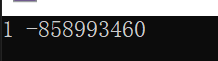因为初始化列表进行初始化的时候是按照成员变量声明的顺序来的，所以_a2先被初始化为随机值，_a1再被初始化为a

展开全文c语言 c++
• 初始化列表是类中构造函数的一部分，用于实例化类中变量时 赋初值。

## 意义

初始化列表是类中构造函数的一部分，用于实例化类中变量时 赋初值。

## 用法

在函数头与函数体之间，用一个‘ ’列出变量，变量后用‘（）’ 给出实例化该变量时要赋予的初值

用例：

class Date {
public:

Date(int year, int month, int day)
:_year(year), _month(month),_day(day)//初始化列表
{
;
}
private:
int _year;
int _month;
int _day;
};

## 与在函数体内初始化的区别

初始化列表 实在实例化，也就是为变量开辟空间后，立刻赋予初始值。

类似于

int a=10;//实例化时赋予初始值

而在构造函数体内初始化 ，则是先为变量开辟空间，后赋予值。

类似于

int a;//先为变量开辟空间
a=10;//后赋予值

## 常见的使用场景

### 1.调用类中 自定义类型成员的构造函数

#include<iostream>
class a {
public:
a(int val,int date) {
_val = val;
}
private:
int _val;
int _date;
};
class  A {
public:
A(int val = 1)
:ma(5,4)
{
_val = val;
}
int _val;
a ma;
};

因为 class a没有默认构造函数，需要主动传参，而构造函数只能在实例化对象的时候调用，并且要先实例化成员变量才会进入class A的构造函数体内，所以，只能使用class A的初始化列表来调用class a的构造函数 初始化A的 成员变量ma。

### 2.类中 const修饰的成员变量

#include<iostream>
class A {
public:
A(int val):_val(val){}
const int _val;
};
int main() {
A a (2);
std::cout << a._val;
return 0;
}

具有常属性的变量需要在实例化时赋初值

### 3.类中 引用类型的成员变量

#include<iostream>
class A {
public:
A(int val):_val(val),mval(_val){}
const int _val;
const int& mval;
};
int main() {
A a (2);
std::cout << a.mval;
return 0;
}

以上这就是初始化列表非常典型的使用场景。

展开全文c++ 开发语言
•c++
• ## C++ 初始化列表

千次阅读 2021-11-24 17:19:53
C++ 提供了初始化列表语法，用来初始化属性 语法：构造函数（）：属性1（值1），属性2（值2）....{ } 1、传统初始化操作 #include <iostream> using namespace std; //初始化列表 class Person { ...c++
• Python是一种非常灵活的语言，可以通过多种方式执行单个任务，例如，可以通过多种方式执行初始化列表。下面本篇文章就来带大家了解几种Python初始化的方法，并比较一下这几种方法的效率，希望对大家有所帮助。下面...
•C++
• 构造函数初始化列表 与普通构造函数的区别 构造函数初始化列表用于在显式初始化类成员。在平常的使用构造函数创建对象时，如果对象中将另一个对象作为成员，那么在调用构造函数之前，编译器会用成员对象的默认构造...c++ 编程语言
• EnumTest-初始化类的类成员对象（初始化列表方式）
• 使用初始化列表的原因         必须使用初始化列表的时候         成员变量的顺序 定义 与其他函数不同，构造函数除了有名字，...c++
• ## c++中的初始化列表详解

万次阅读 多人点赞 2018-05-18 18:15:04
与其他函数不同，构造函数除了有名字，参数列表和函数体之外，还可以有初始化列表初始化列表以冒号开头，后跟一系列以逗号分隔的初始化字段。 class foo { public: foo(string s, int i):name(s), id(i){} ; //...c++ 构造函数
• ## Python初始化列表

万次阅读 多人点赞 2019-04-29 18:17:49
Python初始化列表 下面我们就来介绍在Python中初始化列表（我们创建大小为1000并使用零初始化的列表）的一些方法。 1）方法一：使用for循环和append()函数 arr = [] for i in range(1000): arr.append(0) 2）方法...Python
• C++类和对象——初始化列表、static成员和友元一、再谈构造函数1.1 构造函数整体赋值1.2 初始化列表三级目录 关于C++类和对象的学习 C++类和对象(上)——类的基本概念、类的限定符及封装和类成员函数的this指针 C++...c++ 类和对象 static...